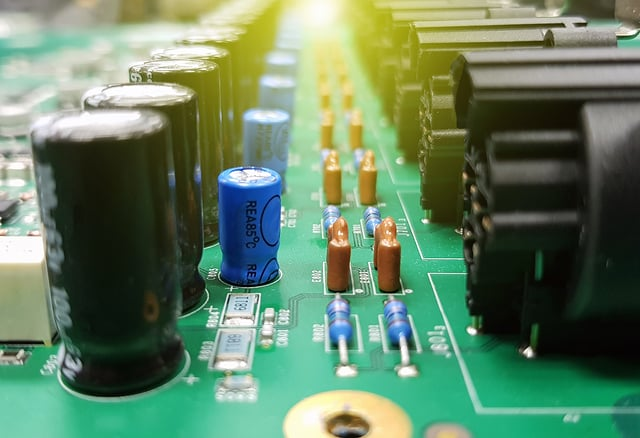# Formulas You’ll Need for the ASVAB Electronics Test

If you are interested in electronics and want a job maintaining and repairing electronic systems such as radios, radars, mine detectors, GPSs, or drones while in the military, you’ll need a good grade on the ASVAB Electronics Test. If you want to achieve this goal, we’re willing to help you! Below, you’ll find a formula chart with all the essential formulas you’ll need to know for acing the ASVAB Electronics Test.

Keep in mind that you won’t be able to use the formula chart during the actual test, but we strongly recommend using it as a guide for reviewing the essential content and while solving the sample problems at Union Test Prep.

Formula Symbols Comment
$$F = k \cdot \frac{q_1 \cdot q_2}{r^2}$$ $$F=\text{Electric Force (N)}$$
$$k = \scriptsize\text{Coulomb's Constant} = 8.988 \cdot 10^9 \frac{Nm^2}{C^2}$$
$$q_n = \text{Charge n (C)}$$
$$r = \text{Distance between charges (m)}$$

$$I = \frac{V}{R} = \frac{P}{V} = \sqrt{\frac{P}{R}} = \frac{V}{Z} = \frac{\Delta Q}{\Delta t}$$ $$I = \text{Current (A) }$$
$$V = \text{Voltage (V)}$$
$$R = \text{Resistance } (\Omega)$$
$$P = \text{Power (W)}$$
$$Z = \text{Impedance } (\Omega)$$
$$\Delta Q = \text{change of Charge (C)}$$
$$\Delta t = \text{change of time (s)}$$

$$I_{eq} = I_1 = I_2 = ... = I_n$$ $$I_{eq} = \text{Equivalent Current (A)}$$
$$I_n = \text{Individual Current (A)}$$
Series Circuit
$$I_{eq} = I_1 + I_2 + ... + I_n$$ $$I_{eq} = \text{Equivalent Current (A)}$$
$$I_n = \text{Individual Current (A)}$$
Parallel Circuit
$$\sum{I_k} = 0$$ $$I_k = \text{Current entering a node (A)}$$
$$V = I\cdot R = \frac{P}{I} = \sqrt{P \cdot R} = I \cdot Z = \frac{E}{Q}$$ $$I = \text{Current (A) }$$
$$V = \text{Voltage (V)}$$
$$R = \text{Resistance } (\Omega)$$
$$P = \text{Power (W)}$$
$$Z = \text{Impedance } (\Omega)$$
$$E = \text{Energy (J)}$$
$$Q = \text{Charge (C)}$$

$$V_{eq} = V_1 + V_2 + ... + V_n$$ $$V_{eq} = \text{Equivalent Voltage (V)}$$
$$V_n = \text{Individual Voltage (V)}$$
Series Circuit
$$V_{eq} = V_1 = V_2 = ... = V_n$$ $$V_{eq} = \text{Equivalent Voltage (V)}$$
$$V_n = \text{Individual Voltage (V)}$$
Parallel Circuit
$$\sum{V_k} = 0$$ $$V_k = \text{Voltage in current loop (V)}$$
$$R = \frac{V}{I} = \frac{P}{I^2} = \frac{V^2}{P}$$ $$I = \text{Current (A)}$$
$$V = \text{Voltage (V)}$$
$$R = \text{Resistance } (\Omega)$$
$$P = \text{Power (W)}$$

$$R = \rho \cdot \frac{L}{A}$$ $$R = \text{Resistance } (\Omega)$$
$$\rho = \text{Resistivity } (\Omega \cdot \text{m})$$
$$L =\text{Length (m)}$$
$$A = \text{Cross-sectional Area (m}^2)$$

$$R_{eq} = R_1 + R_2 + ... + R_n$$ $$R_{eq} = \text{Equivalent Resistance }(\Omega)$$
$$R_n = \text{Individual Resistance }(\Omega)$$
Series Circuit
$$\frac{1}{R_{eq}} = \frac{1}{R_1} + \frac{1}{R_2} + ... + \frac{1}{R_n}$$ $$R_{eq} = \text{Equivalent Resistance }(\Omega)$$
$$R_n = \text{Individual Resistance }(\Omega)$$
Parallel Circuit
$$P = R \cdot I^2 = I \cdot V = \frac{V^2}{R}$$ $$I = \text{Current (A)}$$
$$V = \text{Voltage (V)}$$
$$R = \text{Resistance } (\Omega)$$
$$P = \text{Power (W)}$$

$$C = \frac{Q}{V}$$ $$C = \text{Capacitance (F)}$$
$$Q = \text{Charge (C)}$$
$$V = \text{Voltage (V)}$$

$$\frac{1}{C_{eq}} = \frac{1}{C_1} + \frac{1}{C_2} + .. + \frac{1}{C_n}$$ $$C_{eq} = \text{Equivalent Capacitance (F)}$$
$$C_n = \text{Individual Capacitance (F)}$$
Series Circuit
$$C_{eq} = C_1 + C_2 + .. + C_n$$ $$C_{eq} = \text{Equivalent Capacitance (F)}$$
$$C_n = \text{Individual Capacitance (F)}$$
Parallel Circuit
$$\omega = 2 \pi f$$ $$\omega = \text{angular frequency (rad/s)}$$
$$f = \text{frequency (Hz)}$$
$$\pi \approx 3.14$$

$$X_C = \frac{1}{2 \pi fC}$$ $$X_c = \text{Capacitive Reactance } (\Omega)$$
$$f = \text{frequency (Hz)}$$
$$C = \text{Capacitance (F)}$$
$$\pi \approx 3.14$$